NEET  >  Test: Potential due to an Electric Dipole (NCERT)

# Test: Potential due to an Electric Dipole (NCERT)

Test Description

## 5 Questions MCQ Test Physics Class 12 | Test: Potential due to an Electric Dipole (NCERT)

Test: Potential due to an Electric Dipole (NCERT) for NEET 2023 is part of Physics Class 12 preparation. The Test: Potential due to an Electric Dipole (NCERT) questions and answers have been prepared according to the NEET exam syllabus.The Test: Potential due to an Electric Dipole (NCERT) MCQs are made for NEET 2023 Exam. Find important definitions, questions, notes, meanings, examples, exercises, MCQs and online tests for Test: Potential due to an Electric Dipole (NCERT) below.
Solutions of Test: Potential due to an Electric Dipole (NCERT) questions in English are available as part of our Physics Class 12 for NEET & Test: Potential due to an Electric Dipole (NCERT) solutions in Hindi for Physics Class 12 course. Download more important topics, notes, lectures and mock test series for NEET Exam by signing up for free. Attempt Test: Potential due to an Electric Dipole (NCERT) | 5 questions in 5 minutes | Mock test for NEET preparation | Free important questions MCQ to study Physics Class 12 for NEET Exam | Download free PDF with solutions
 1 Crore+ students have signed up on EduRev. Have you?
Test: Potential due to an Electric Dipole (NCERT) - Question 1

### The electric field and the potential of an electric dipole vary with distance r as

Detailed Solution for Test: Potential due to an Electric Dipole (NCERT) - Question 1

Electric field and electric potential at a general point at a distance r from the centre of the dipole is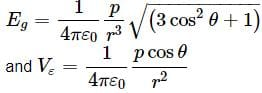Test: Potential due to an Electric Dipole (NCERT) - Question 2

### An electric dipole is placed at the centre of a hollow conducting sphere. Which of the following is correct?

Detailed Solution for Test: Potential due to an Electric Dipole (NCERT) - Question 2

When electric dipole is held in the sphere, electric field is not zero anywhere on the sphere. However, net electric flux through the sphere is zero.

Test: Potential due to an Electric Dipole (NCERT) - Question 3

### Which of the following is not true?

Detailed Solution for Test: Potential due to an Electric Dipole (NCERT) - Question 3

The electric dipole potential varies as 1/r at large distance is not true.

Test: Potential due to an Electric Dipole (NCERT) - Question 4

The distance between H + and Cl − ions in HCl molecules is 1.38 Å. The potential due to this dipole at a distance of 10Å on the axis of dipole is

Detailed Solution for Test: Potential due to an Electric Dipole (NCERT) - Question 4

Here, 2a = 1.38 × 10−10m, r = 10 × 10−10 m charge, q = 1.6 × 10−19C
As potential,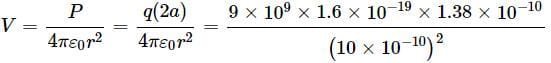Test: Potential due to an Electric Dipole (NCERT) - Question 5

Four points a, b, c and d are set at equal distance from the centre of a dipole as shown in the figure.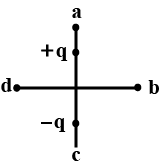The magnitudes of electrostatic potential Va, Vb, Vc and Vd would satisfy the following relation

Detailed Solution for Test: Potential due to an Electric Dipole (NCERT) - Question 5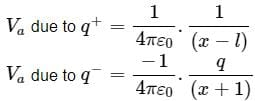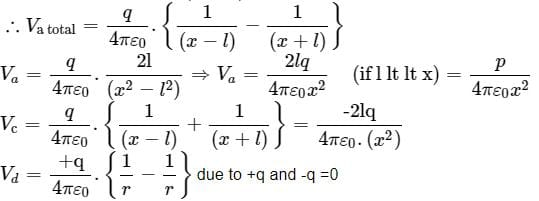Vb = 0
∴ Va = Vc > Vb = Vd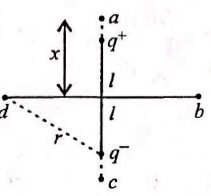## Physics Class 12

157 videos|452 docs|213 tests
 Use Code STAYHOME200 and get INR 200 additional OFF Use Coupon Code
Information about Test: Potential due to an Electric Dipole (NCERT) Page
In this test you can find the Exam questions for Test: Potential due to an Electric Dipole (NCERT) solved & explained in the simplest way possible. Besides giving Questions and answers for Test: Potential due to an Electric Dipole (NCERT), EduRev gives you an ample number of Online tests for practice

## Physics Class 12

157 videos|452 docs|213 tests

### How to Prepare for NEET

Read our guide to prepare for NEET which is created by Toppers & the best Teachers